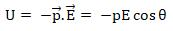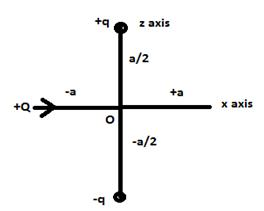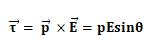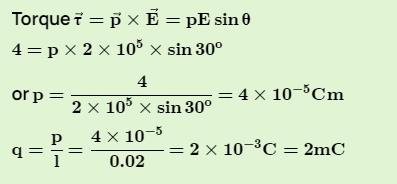Courses

# Test: Electric Dipole

## 10 Questions MCQ Test Physics Class 12 | Test: Electric Dipole

Description
This mock test of Test: Electric Dipole for JEE helps you for every JEE entrance exam. This contains 10 Multiple Choice Questions for JEE Test: Electric Dipole (mcq) to study with solutions a complete question bank. The solved questions answers in this Test: Electric Dipole quiz give you a good mix of easy questions and tough questions. JEE students definitely take this Test: Electric Dipole exercise for a better result in the exam. You can find other Test: Electric Dipole extra questions, long questions & short questions for JEE on EduRev as well by searching above.
QUESTION: 1

### Which of the following molecule has permanent dipole moment?​

Solution:

Ionic behavior implies dipole moment.

HCl is an ionic compound (H+Cl-)
CO2, CH4 and BF3  is a covalent compound

QUESTION: 2

### A dipole is said to be in stable equilibrium when angle between electric field and dipole moment is:​

Solution:Stable equilibrium is attained when potential energy of the dipole system is minimum which is possible at an inclination of 0°.

QUESTION: 3

### What is the angle between the electric dipole moment and the electric field on the equatorial line of the dipole?

Solution:

On equatorial line, the direction of electric field is reversed to that of angle of axial line. Therefore, the angle between dipole moment and electric field is 180°.

QUESTION: 4

An electric dipole is

Solution:

An electric dipole is a separation of positive and negative charges. The simplest example of this is a pair of electric charges of equal magnitude but opposite sign, separated by some (usually small) distance. A permanent electric dipole is called an electret.

QUESTION: 5

How does electric field strength change with distance from a short dipole?

Solution:

Electric Field Intensity due to a short dipole is given by

E = K2P/r^3

where K is the proportionality constant

P is the electric dipole moment

So electric field intensity is inversely proportional to r^3

QUESTION: 6

An electric dipole consists of a positive and negative charge of 4 µC. Each placed at a distance of 5 mm. The dipole moment for the system is

Solution:

q= 4μc = 4 x 10-6c
2l = 5mm = 5 x 10-3m
Dipole moment  P = q.2l
= 4 x 10-6 x 5 x 10-3
= 20 x 10-9
= 2 x 10 x 10-9
= 2 x 10-8 c.m

its direction is toward the positive charge.

QUESTION: 7

Which of the following quantity is a scalar?

Solution:

Electric flux is a scalar quantity. It is a scalar because it is the dot product of two vector quantities, electric field and the perpendicular differential area.

QUESTION: 8

For forming an electric dipole between two point charges separated by a small distance, the two point charges are of

Solution:

By definition of Electric Dipole

QUESTION: 9

Positive and negative point charges of equal magnitude are kept at locations ( 0,0,a/2) and (0,0,-a/2) respectively. The work done by the electric field when another positive point charge is moved from (-a,0,0) to (0,a,0) is

Solution:+Q charge is moving on equatorial line of dipole as shown in figure, since on equatorial line V=0,
Hence the potential difference is zero. So, W=0

QUESTION: 10

An electric dipole is placed at an angle of 30° with an electric field intensity 2 × 105 N/C. It experiences a torque equal to 4 N m. The charge on the dipole, if the dipole length is 2 cm, is

Solution:

Torque on a dipole kept in external electric field is given by the expression,